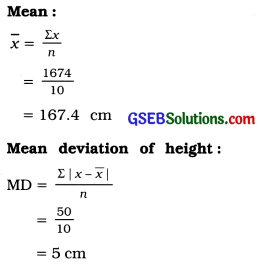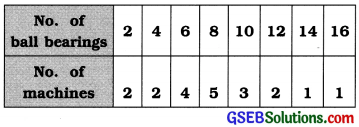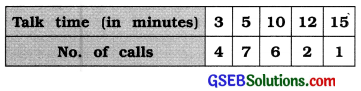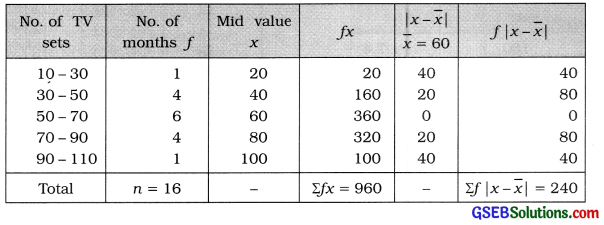# GSEB Solutions Class 11 Statistics Chapter 4 Measures of Dispersion Ex 4.3

Gujarat Board Statistics Class 11 GSEB Solutions Chapter 4 Measures of Dispersion Ex 4.3 Textbook Exercise Questions and Answers.

## Gujarat Board Textbook Solutions Class 11 Statistics Chapter 4 Measures of Dispersion Ex 4.3

Question 1.
The measurements of height (in centimeters) of 10 soldiers are given below:Find the mean deviation of the heights of the soldiers.
Here, n = 10

 Height (in cm) | x – x̄ | x̄  = 167.4 160 7.4 175 7.6 158 9.4 165 2.4 170 2.6 166 1.4 173 5.6 176 8.6 163 4.4 168 0.6 Σx = 1674 Σ |x – x̄| = 50.0Question 2.
The distribution of number of ball bearings used in machines of a factory is given below. Calculate the mean deviation and coefficient of mean deviation of number of ball bearings per machine.Mean:
x̄ = $$\frac{\Sigma f x}{n}=\frac{160}{20}$$ = 8 ball bearing

Mean deviation of numbers of ball bearing:
MD = $$\frac{\Sigma f|x-\bar{x}|}{n}$$
= $$\frac{56}{20}$$ = 28 ball bearing

Coefficient Mean deviation of ball bearing:
Coefficient of mean deviation = $$\frac{\mathrm{MD}}{\bar{x}}$$
= $$\frac{2.8}{8}$$ = 0.35Question 3.
Find the mean deviation and the coefficient of mean deviation of the distribution of talk time (in minutes) per call.Coefficient of Mean deviation of talk time : Coefficient of Mean deviation = $$\frac{\mathrm{MD}}{\bar{x}}=\frac{3.33}{7.3}$$ = 0.46

Question 4.
Find the mean deviation and coefficient of mean deviation of number of TV sets using the following frequency distribution of TV sets sold in last 16 months in a town:Mean:
x̄ = $$\frac{\Sigma f x}{n}=\frac{960}{16}$$ = 60 TV sets

Mean deviation of monthly sales of TV sets:
MD = $$\frac{\Sigma f|x-\bar{x}|}{n}=\frac{240}{16}$$ = 15 TV sets

Coefficient of Mean deviation of monthly sales of TV sets:
Coefficient of mean deviation = $$\frac{M D}{\bar{x}}=\frac{15}{60}$$ = 0.25Question 5.
There are 50 boxes containing different number of units of an item in a factory. Find the mean deviation of number of units per box using the following distribution of the units:x̄ = $$\frac{\Sigma f x}{n}=\frac{1670}{50}$$ = 33.4 units
MD = $$\frac{\Sigma f|x-\bar{x}|}{n}=\frac{659.2}{50}$$ = 13.18 units